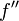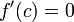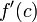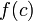# Second derivative test

Jump to: navigation, search
This article describes a test that can be used to determine whether a point in the domain of a function gives a point of local, endpoint, or absolute (global) maximum or minimum of the function, and/or to narrow down the possibilities for points where such maxima or minima occur.
View a complete list of such tests

## Statement

### What this test is for

This test is a partial test (i.e., it may be inconclusive) for determining whether a given critical point for a function is a point of local minimum, point of local maximum, or neither.

### What the test says

Suppose$f$ is a function and$c$ is a point in the interior of the domain of$f$, i.e.,$f$ is defined on some open interval containing$c$. Suppose, further, that$f''$, i.e., the second derivative of$f$, exists at$c$. Suppose also that$f'(c)=0$, so$c$ is a critical point for$f$. Then:

Hypothesis on$f'(c)$ Hypothesis on$f''(c)$ Conclusion$f'(c) =0$$f''(c) < 0$$f$ attains a strict local maximum value at$c$ (the value is$f(c)$)$f'(c) = 0$$f''(c) > 0$$f$ attains a strict local minimum value at$c$ (the value is$f(c)$)$f'(c) = 0$$f''(c) = 0$ The test is inconclusive.$f$ may attain a local maximum value, a local minimum value, have a point of inflection, or have some different behavior at the point$c$.

### Relation with critical points

The second derivative test is specifically used only to determine whether a critical point where the derivative is zero is a point of local maximum or local minimum. Note in particular that:

• For the other type of critical point, namely that where$f'$ is undefined, the second derivative test cannot be used.
• Since point of local extremum implies critical point, we don't have to worry about points that are not critical points -- none of them will give local extrema.

## Facts used

1. One-sided version of second derivative test

## Proof

The proof follows directly from Fact (1).

## Relation with other tests

### Other tests to determine whether critical points give local extreme values

Test Quick description of how it differs from the second derivative test Relation with second derivative test
one-sided version of second derivative test We compute one-sided second derivatives instead of the usual two-sided second derivative. It is somewhat stronger and more general, i.e., it is applicable and conclusive in a somewhat wider range of circumstances.
first derivative test We compute the sign of the first derivative instead of the second derivative and perform the sign computation on the immediate left and immediate right of the point rather than at the point. It is stronger: second derivative test operates via first derivative test, second derivative test is not stronger than first derivative test
higher derivative test This begins with the second derivative test but computes higher derivatives at the point if the second derivative turns out to be zero, looking for the lowest order nonzero-valued derivative at the point. It is conclusive in a somewhat wider range of circumstances. Note that the test begins the same way as the second derivative test, and we use higher derivatives only if necessary.
One-sided derivative test We compute the signs of one-sided first derivatives at the point. Not directly comparable.

## Requirement of twice differentiability

The second derivative test can be applied at a critical point$c$ for a function$f$ only if$f$ is twice differentiable at$c$. This in particular forces$f$ to be once differentiable around$c$.

However, the test does not require the second derivative$f''$ to be defined around$c$ or to be continuous at$c$.

## Ease of use

The second derivative test is strictly less powerful than the first derivative test, so why is it ever used? The main reason is that in cases where it is conclusive, the second derivative test is often easier to apply. This, in turn, is because the second derivative test only requires the computation of formal expressions for derivatives and evaluation of the signs of these expressions at a point rather than on an interval. Evaluation at a point often requires less symbolic/algebraic manipulation.

## Inconclusive and conclusive cases

### The test can never be conclusive about the absence of local extrema

The first derivative test can sometimes conclusively establish that a given critical point is not a point of local extremum. The second derivative test can never conclusively establish this. It can only conclusively establish affirmative results about local extrema.

### Inconclusive cases

The second derivative test is inapplicable or inconclusive in the following situations. We denote the function by$f$ and the critical point by$c$:

What problem do we run into? What kind of trouble do we have? Can we use the first derivative test? Link to example/explanation Remedy that may work (other than reverting to the first derivative test)
The critical point is of the type where$f'$ is undefined We cannot take the second derivative, because even the first derivative doesn't exist. We may or may not be able to use the first derivative test. It is possible to construct examples of (local min + conclusive first derivative test), (local max + conclusive first derivative test), (neither + conclusive first derivative test), (local min + inconclusive first derivative test), (local max + inconclusive first derivative test), (neither + inconclusive first derivative test) second derivative test fails for function at critical point where it is not differentiable$f'(c) = 0$ but$f''(c)$ is undefined We cannot take the second derivative at the critical point. We may or may not be able to use the first derivative test. It is possible to construct examples of (local min + conclusive first derivative test), (local max + conclusive first derivative test), (neither + conclusive first derivative test), (local min + inconclusive first derivative test), (local max + inconclusive first derivative test), (neither + inconclusive first derivative test) second derivative test fails for function at critical point where it is differentiable but not twice differentiable one-sided version of second derivative test: If the one-sided derivatives of$f'$ exist at$c$, then we can try checking that both one-sided derivatives of$f'$ have the stipulated sign for$f''$.$f'(c) = 0$ and$f''(c) = 0$ We are in the inconclusive case of the test as stated. We may or may not be able to use the first derivative test. It is possible to construct examples of (local min + conclusive first derivative test), (local max + conclusive first derivative test), (neither + conclusive first derivative test), (local min + inconclusive first derivative test), (local max + inconclusive first derivative test), (neither + inconclusive first derivative test) second derivative test is inconclusive for function at critical point where second derivative is zero higher derivative test